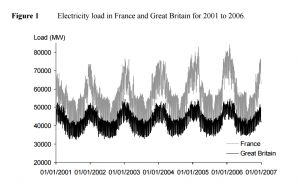8
Jun 17

## Autoregressive processes

Autoregressive processes: going from the particular to the general is the safest option. Simple observations are the foundation of any theory.

## IntuitionFigure 1. Electricity load in France and Great Britain for 2001 to 2006

If you have only one variable, what can you regress it on? Only on its own past values (future values are not available at any given moment). Figure 1 on electricity demand from a paper by J.W. Taylor illustrates this. A low value of electricity demand, say, in summer last year, will drive down its value in summer this year. Overall, we would expect the electricity demand now to depend on its values in the past 12 months. Another important observation from this example is that probably this time series is stationary.

## AR(p) model

We want a definition of a class of stationary models. From this example we see that excluding the time trend increases chances of obtaining a stationary process. The idea to regress the process on its own past values is realized in

(1)$y_t=\mu+\beta_1y_{t-1}+...+\beta_py_{t-p}+u_t.$

Here$p$ is some positive integer. However, both this example and the one about random walk show that some condition on the coefficients$\mu,\beta_1,...,\beta_p$ will be required for (1) to be stationary. (1) is called an autoregressive process of order$p$ and denoted AR(p).

Exercise 1. Repeat calculations on AR(1) process to see that in case$p=1$ for (1) the stability condition$|\beta_1|<1$ is sufficient for stationarity (that is, the coefficient$\mu$ has no impact on stationarity).

Question. How does this stability condition generalize to AR(p)?

## Characteristic polynomial

Denote$L$ the lag operator defined by$Ly_t=y_{t-1}$. More generally, its powers are defined by$L^ky_t=y_{t-k}$. Then (1) can be rewritten as$y_t=\mu+\beta_1Ly_t+...+\beta_pL^py_t+u_t.$

Whoever first did this wanted to solve the equation for$y_t$. Sending all terms containing$y_t$ to the left we have$y_t-(\beta_1Ly_t+...+\beta_pL^py_t)=\mu+u_t.$

The identity operator is defined by$Iy_t=y_t$, so$y_t=Iy_t$. Factoring out$y_t$ we get

(2)$(I-\beta_1L-...-\beta_pL^p)y_t=\mu+u_t.$

Finally, formally solving for$y_t$ we have

(3)$y_t=(I-\beta_1L-...-\beta_pL^p)^{-1}(\mu+u_t).$

Definition 1.  In$I-\beta_1L-...-\beta_pL^p$ replace the identity by 1 and powers of the lag operator by powers of a real number$x$ to obtain the definition of the characteristic polynomial:

(3)$p(x)=1-\beta_1x-...-\beta_px^p$.$p(x)$ is a polynomial of degree$p$ and by the fundamental theorem of algebra has$p$ roots.

Definition 2. We say that model (1) is stable if its characteristic polynomial (3) has roots outside the unit circle, that is, the roots are larger than 1 in absolute value.

Under this stability condition the passage from (2) to (3) can be justified. For AR(1) process this actually has been done.

Example 1. In case of a first-order process,$p(x)=1-\beta_1x$ has one root$x=1/\beta_1$ which lies outside the unit circle exactly when$|\beta_1|<1.$

Example 2. In case of a second-order process,$p(x)$ has two roots. If both of them are larger than 1 in absolute value, then the process is stable. The formula for the roots of a quadratic equation is well-known but stating it here wouldn't add much to what we know. Most statistical packages, including Stata, have procedures for checking stability.

Remark. Hamilton uses a different definition of the characteristic polynomial (linked to vector autoregressions), that's why in his definition the roots of the characteristic equation should lie inside the unit circle.